Back

Quiz

1. A resultant force causes a body to accelerate. what is equal to the resultant force

The accelaration of the body per unit mass

The change in kinetic energy of the body per unit time

The change in momentum of the body per unit time

The change in velocity of the body per unit time

2. A solid sphere, which is less dense than water, is held completely immersed in water a few metres below the surface. The density of the water is uniform.

The sphere is released. Immediately after release, the sphere rises.

Which row correctly describes the changes in the upthrust on the sphere and the resultant force on the sphere?

upthrust on the sphere : constant ; resultant force on the sphere: increasing

upthrust on the sphere : constant ; resultant force on the sphere: decreasing

upthrust on the sphere : decreasing ; resultant force on the sphere: increasing

upthrust on the sphere : decreasing ; resultant force on the sphere: decreasing

3. A piston in a gas supply pump has an area of 500 cm 2 and it moves a distance of 30 cm.

The pump moves the gas against a fixed pressure of 4000 Pa.

How much work is done by the piston?

60 J

6.0 × 10 3 J

6.0 × 10 5 J

6.0 × 10 7 J

4. A cathode-ray oscilloscope (CRO) is used to display a wave of frequency 5.0 kHz. The display is shown.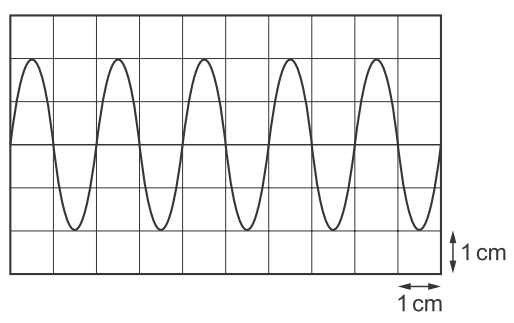What is the time-base setting of the CRO?

10 µs cm -1

100 µs cm -1

10 ms cm -1

100 ms cm -1

5. In an experiment to demonstrate a stationary wave, two microwave transmitters, emitting waves wavelength 4 cm, re set facing each other, as shown.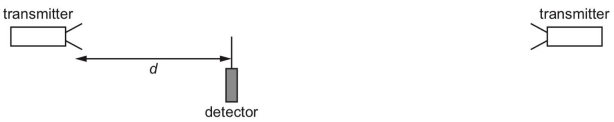A detector is moved along a straight line between the transmitters. It detects positions of maximum and minimum signal. The detector is a distance d from the left-hand transmitter.

Assume that both transmitters are at antinodes of the stationary wave.

Which row gives a value of d for a maximum and for a minimum?

value of d for a maximum/cm: 46; value of d for a minimum/cm: 48

value of d for a maximum/cm: 47; value of d for a minimum/cm: 48

value of d for a maximum/cm: 48; value of d for a minimum/cm: 47

value of d for a maximum/cm: 49; value of d for a minimum/cm: 47

6. Diagram shows a solid cube with weight W and sides of length L. It is supported by a frictionless spindle that passes through the centres of two opposite vertical faces. One of these faces is shaded.Spindle is now removed and replaced at a distance L / 4 to the right of its original position.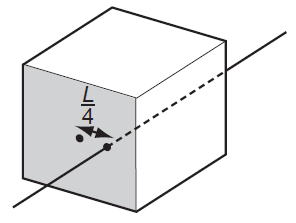When viewing the shaded face, what is torque of the couple that will now be needed to stop the cube from toppling?

WL2 anticlockwise

WL2 clockwise

WL4 anticlockwise

WL4 clockwise

7. A pipe is closed at one end and contains gas, trapped by column of water.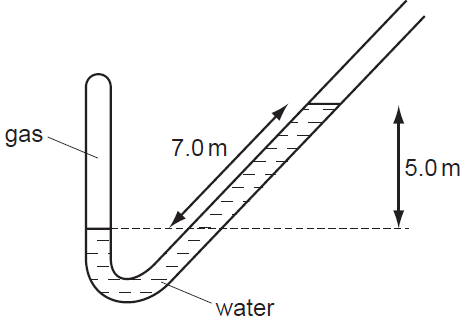Atmospheric pressure is 1.0 × 105 Pa. Density of water is 1000 kg m–3.

What is the pressure of the gas? (Use g = 10 m s–2.)

0.3 × 105 Pa

0.5 × 105 Pa

1.5 × 105 Pa

1.7 × 105 Pa

8. Monochromatic plane wave of speed c and wavelength λ is diffracted at small aperture.

Diagram illustrates successive wavefronts.After what time will some portion of wavefront XY reach point P?

3λ / 2c

2λ / c

3λ / c

4λ / c

9. Nuclear decay is both spontaneous and random in nature

Which row gives the correct experimental evidence for these properties?

spontaneous nature of decay: the decay rate is not affected by pressure; random nature of decay: the decay rate is not affected by temperature

the decay rate is not affected by pressure; the rate at which radiation is received at a counter fluctuates

the decay rate is not affected by temperature; the decay rate is not affected by pressure

the rate at which radiation is received at a counter fluctuates; the decay rate is not affected by pressure

10. A single horizontal force F is applied to a block X which is in contact with a separate block Y as shown.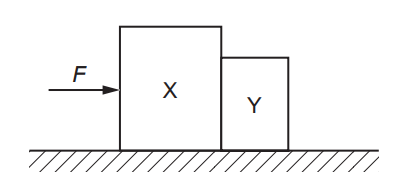The blocks remain in contact as they accelerate along a horizontal frictionless surface. Air resistance is negligible. X has a greater mass than Y.
Which statement is correct?

The acceleration of X is equal to force F divided by the mass of X.

The force that X exerts on Y is equal to F.

The force that X exerts on Y is less than F.

The force that X exerts on Y is less than the force that Y exerts on X.

`Try to get the maximum point, 10, in every section. The sections with points lower than 10 are your weaknesses.`